Smartick is an advanced online program that teaches kids math and coding in only 15 min. a day

Dec19

# Locating Numbers on the Number Line

What is a number line? Can all numbers be found on it? How is it used? Here, we’ll explain it to you.

#### To begin, we are going to review what the number line is.

A straight line is an infinite arrangement of points in the same direction. Thus, the number line is a line in which you can assign a value of a real number to each of your points.

Now that we know what a number line is, we can see, with different examples that use natural numbers, integers, and rational numbers, how to locate the different numbers on the number line.

1. We’ll begin with the most straightforward, the natural numbers (N), which are those used to count.

To begin, we mark a point on the number line, which we call 0 and we divide the rest of the line into segments of the same length. Each one represents a unit, which separates one number from the next, as shown: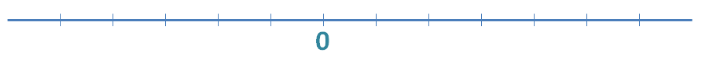A line divided into segments of the same length with a point that we named 0.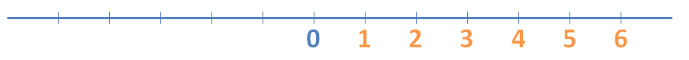A line divided into segments of the same size with the natural numbers placed on each mark to the right of point 0.

1. The integers (Z) represent the natural numbers and the same intervals in the opposite direction from point 0. Thus: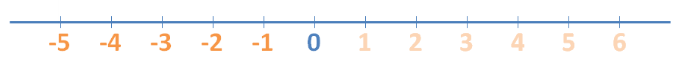A line divided into unit segments with negative integers placed to mirror the numbers to the left of point 0.

1. Following are the rational numbers (Q), which include the integers and natural numbers and also all of the numbers that can be expressed in fraction form.

It’s very easy: the denominator of the fraction expresses how many equal parts the unit (1) is divided into, and the numerator tells us how many of the parts to count over to get to the location on the number line.

In addition, if the number is positive, it will be located to the right of the 0 and if it is negative, on the left. Thus: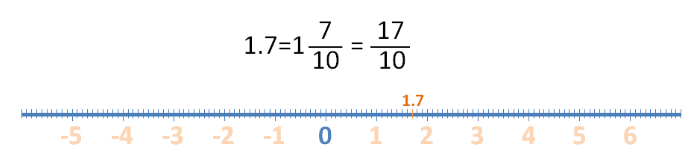A line divided into segments of 0.1 in which the number is located at 1.7.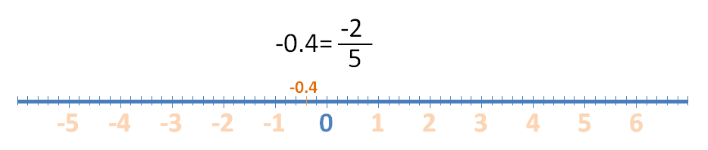A line divided into segments of 0.1 in which the number is located at -0.4.

To represent the rest of the real numbers (R) is more complicated and will be worked on during the senior year of high school. A good teaching resource for square roots is the use of triangles and circles, including the Pythagorean Theorem and property of the circumference. As shown: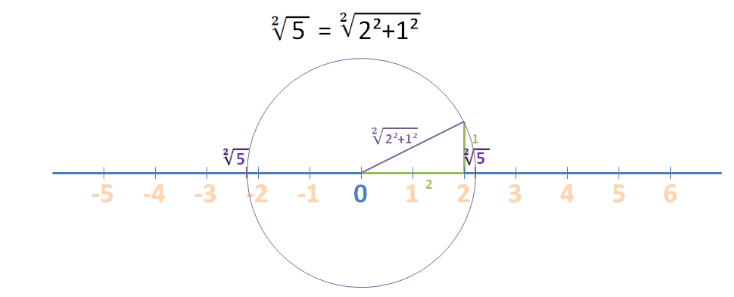A right triangle with known leg lengths determines a hypotenuse that is of equal length to the number you want to place on the number line.

Finally, emphasize the importance of using and working with the number line in primary education, as it is a tool that allows us to relate to elements of geometry and arithmetic in order to ease the comprehension of the concept of a number.